Warning

This documents an unmaintained version of NetworkX. Please upgrade to a maintained version and see the current NetworkX documentation.

# local_node_connectivity¶

local_node_connectivity(G, s, t, flow_func=None, auxiliary=None, residual=None, cutoff=None)[source]

Computes local node connectivity for nodes s and t.

Local node connectivity for two non adjacent nodes s and t is the minimum number of nodes that must be removed (along with their incident edges) to disconnect them.

This is a flow based implementation of node connectivity. We compute the maximum flow on an auxiliary digraph build from the original input graph (see below for details).

Parameters: G (NetworkX graph) – Undirected graph s (node) – Source node t (node) – Target node flow_func (function) – A function for computing the maximum flow among a pair of nodes. The function has to accept at least three parameters: a Digraph, a source node, and a target node. And return a residual network that follows NetworkX conventions (see maximum_flow() for details). If flow_func is None, the default maximum flow function (edmonds_karp()) is used. See below for details. The choice of the default function may change from version to version and should not be relied on. Default value: None. auxiliary (NetworkX DiGraph) – Auxiliary digraph to compute flow based node connectivity. It has to have a graph attribute called mapping with a dictionary mapping node names in G and in the auxiliary digraph. If provided it will be reused instead of recreated. Default value: None. residual (NetworkX DiGraph) – Residual network to compute maximum flow. If provided it will be reused instead of recreated. Default value: None. cutoff (integer, float) – If specified, the maximum flow algorithm will terminate when the flow value reaches or exceeds the cutoff. This is only for the algorithms that support the cutoff parameter: edmonds_karp() and shortest_augmenting_path(). Other algorithms will ignore this parameter. Default value: None. K – local node connectivity for nodes s and t integer

Examples

This function is not imported in the base NetworkX namespace, so you have to explicitly import it from the connectivity package:

>>> from networkx.algorithms.connectivity import local_node_connectivity


We use in this example the platonic icosahedral graph, which has node connectivity 5.

>>> G = nx.icosahedral_graph()
>>> local_node_connectivity(G, 0, 6)
5


If you need to compute local connectivity on several pairs of nodes in the same graph, it is recommended that you reuse the data structures that NetworkX uses in the computation: the auxiliary digraph for node connectivity, and the residual network for the underlying maximum flow computation.

Example of how to compute local node connectivity among all pairs of nodes of the platonic icosahedral graph reusing the data structures.

>>> import itertools
>>> # You also have to explicitly import the function for
>>> # building the auxiliary digraph from the connectivity package
>>> from networkx.algorithms.connectivity import (
...     build_auxiliary_node_connectivity)
...
>>> H = build_auxiliary_node_connectivity(G)
>>> # And the function for building the residual network from the
>>> # flow package
>>> from networkx.algorithms.flow import build_residual_network
>>> # Note that the auxiliary digraph has an edge attribute named capacity
>>> R = build_residual_network(H, 'capacity')
>>> result = dict.fromkeys(G, dict())
>>> # Reuse the auxiliary digraph and the residual network by passing them
>>> # as parameters
>>> for u, v in itertools.combinations(G, 2):
...     k = local_node_connectivity(G, u, v, auxiliary=H, residual=R)
...     result[u][v] = k
...
>>> all(result[u][v] == 5 for u, v in itertools.combinations(G, 2))
True


You can also use alternative flow algorithms for computing node connectivity. For instance, in dense networks the algorithm shortest_augmenting_path() will usually perform better than the default edmonds_karp() which is faster for sparse networks with highly skewed degree distributions. Alternative flow functions have to be explicitly imported from the flow package.

>>> from networkx.algorithms.flow import shortest_augmenting_path
>>> local_node_connectivity(G, 0, 6, flow_func=shortest_augmenting_path)
5


Notes

This is a flow based implementation of node connectivity. We compute the maximum flow using, by default, the edmonds_karp() algorithm (see: maximum_flow()) on an auxiliary digraph build from the original input graph:

For an undirected graph G having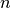nodes and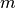edges we derive a directed graph H with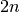nodes and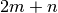arcs by replacing each original node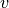with two nodes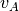,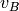linked by an (internal) arc in H. Then for each edge (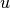,) in G we add two arcs (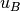,) and (,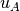) in H. Finally we set the attribute capacity = 1 for each arc in H  .

For a directed graph G havingnodes andarcs we derive a directed graph H withnodes and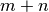arcs by replacing each original nodewith two nodes,linked by an (internal) arc (,) in H. Then for each arc (,) in G we add one arc (,) in H. Finally we set the attribute capacity = 1 for each arc in H.

This is equal to the local node connectivity because the value of a maximum s-t-flow is equal to the capacity of a minimum s-t-cut.

local_edge_connectivity(), node_connectivity(), minimum_node_cut(), maximum_flow(), edmonds_karp(), preflow_push(), shortest_augmenting_path()